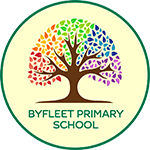# Maths

We teach Maths using the Maths No Problem scheme, an approach to teaching maths developed in Singapore. Problem solving, fluency and relational understanding are at the heart of the scheme. It uses the Concrete Pictorial Abstract (CPA) approach and allows pupils to spend enough time to fully explore a topic, reinforcing it with practice, before moving onto the next one. All ideas are built on previous knowledge and pupils have ample opportunity to develop relationships between topics.

Lessons typically are broken into four parts:

1. Anchor Task – the entire class spends time on a question guided by the teacher. The children are encouraged during this time to think of as many ways as possible to solve the question as possible.
2. New Learning – the teacher introduces and explains the new learning for the lesson.
3. Guided Practice – children practice new learning in groups, pairs or individually guided by the teacher.
4. Independent Practice – practice on your own. Once children have mastered the concept they use their reasoning and problem-solving skills to develop their depth of learning.

Key points:

• A highly effective approach to teaching maths based on research and evidence
• Builds students’ mathematical fluency without the need for rote learning
• Introduces new concepts using Bruner’s Concrete Pictorial Abstract (CPA) approach
• Pupils learn to think mathematically as opposed to reciting formulas they don’t understand
• Teaches mental strategies to solve problems such as drawing a bar model

Find out more at the Maths No Problem website.

Have a look at the programmes of study for each year group: